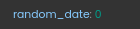# How to calculate the difference between the number of years from the current date?

How to calculate the difference between the number of years from the current date?

17 Likes

We can use YEARSDIFFFROMNOW(date,dateFormat) this function to achieve this.

27 Likes

@Nikhil.Dhanda What is the format of the output for this function?

5 Likes

@VivekBhardwaj It will give the output in Number.

In the configuration I have simply execute a control copy task to copy the output on a temporary path. As shown below -

And the output Will be this -

22 Likes

This gives output in number of years?
What if we need the difference in number of days, months, etc ?

5 Likes

Yes, it gives output in Number of years.

For that there are different functions –

For finding difference in Days - DAYSDIFFFROMNOW(date,dateFormat)

For finding difference in Months - MONTHSDIFFFROMNOW(date,dateFormat)

22 Likes

These are the functions to find the difference from the Current date only.

21 Likes

And the configurations on the developer portal is same for all these functions?
Also, if the difference is less then 1, does it show 0 or actual difference in decimal?

5 Likes

Configuration on the developer portal will be same for all these functions, just you have to change function name in the Task.

23 Likes

Also, if the difference is less then 1, does it show 0 or actual difference in decimal?

6 Likes

If the difference is less than 1 … then it will show 0 as the output.

For this case, I have entered the date of 2023 itself.

and the output will be-24 Likes